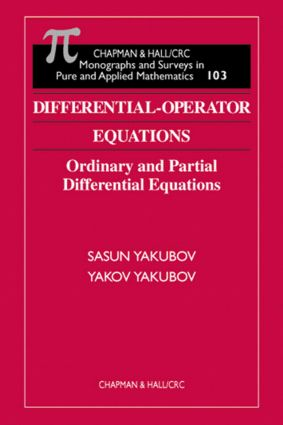# Differential-Operator Equations

## Ordinary and Partial Differential Equations, 1st Edition

Chapman and Hall/CRC

576 pages

##### Purchasing Options:\$ = USD
Hardback: 9781584881391
pub: 1999-11-24
SAVE ~\$60.00
\$300.00
\$240.00
x

FREE Standard Shipping!

### Description

The theory of differential-operator equations is one of two modern theories for the study of both ordinary and partial differential equations, with numerous applications in mechanics and theoretical physics. Although a number of published works address differential-operator equations of the first and second orders, to date none offer a treatment of the higher orders.

In Differential-Operator Equations, the authors present a systematic treatment of the theory of differential-operator equations of higher order, with applications to partial differential equations. They construct a theory that allows application to both regular and irregular differential problems. In particular, they study problems that cannot be solved by various known methods and irregular problems not addressed in existing monographs. These include Birkhoff-irregularity, non-local boundary value conditions, and non-smoothness of the boundary of the domains.

Among this volume's other points of interest are:

• The Abel basis property of a system of root functions

• Irregular boundary value problems

• The theory of hyperbolic equations in Gevrey space

• The theory of boundary value problems for elliptic differential equations with a parameter

• ### Table of Contents

Introduction

Auxiliary Results

Completeness and the Abel Basis Property of a System of Root Vectors

Principally Boundary Value Problems for Ordinary Differential Equations with a Polynomial Parameter

Principally Regular Elliptic Boundary Value Problems with a Polynomial Parameter

Elliptic Differential-Operator Equations

Hyperbolic Differential-Operator Equations

Parabolic Differential-Operator Equations

Well-Posed Problems for Partial Differential Equations

Problems

Reference Notes

References

List of Notations

Subject Index

Author Index

### Subject Categories

##### BISAC Subject Codes/Headings:
MAT007000
MATHEMATICS / Differential Equations
MAT037000
MATHEMATICS / Functional Analysis
SCI040000
SCIENCE / Mathematical Physics